# 教你用js开发【风来之国】游戏之地图篇！入门教程，大佬勿进~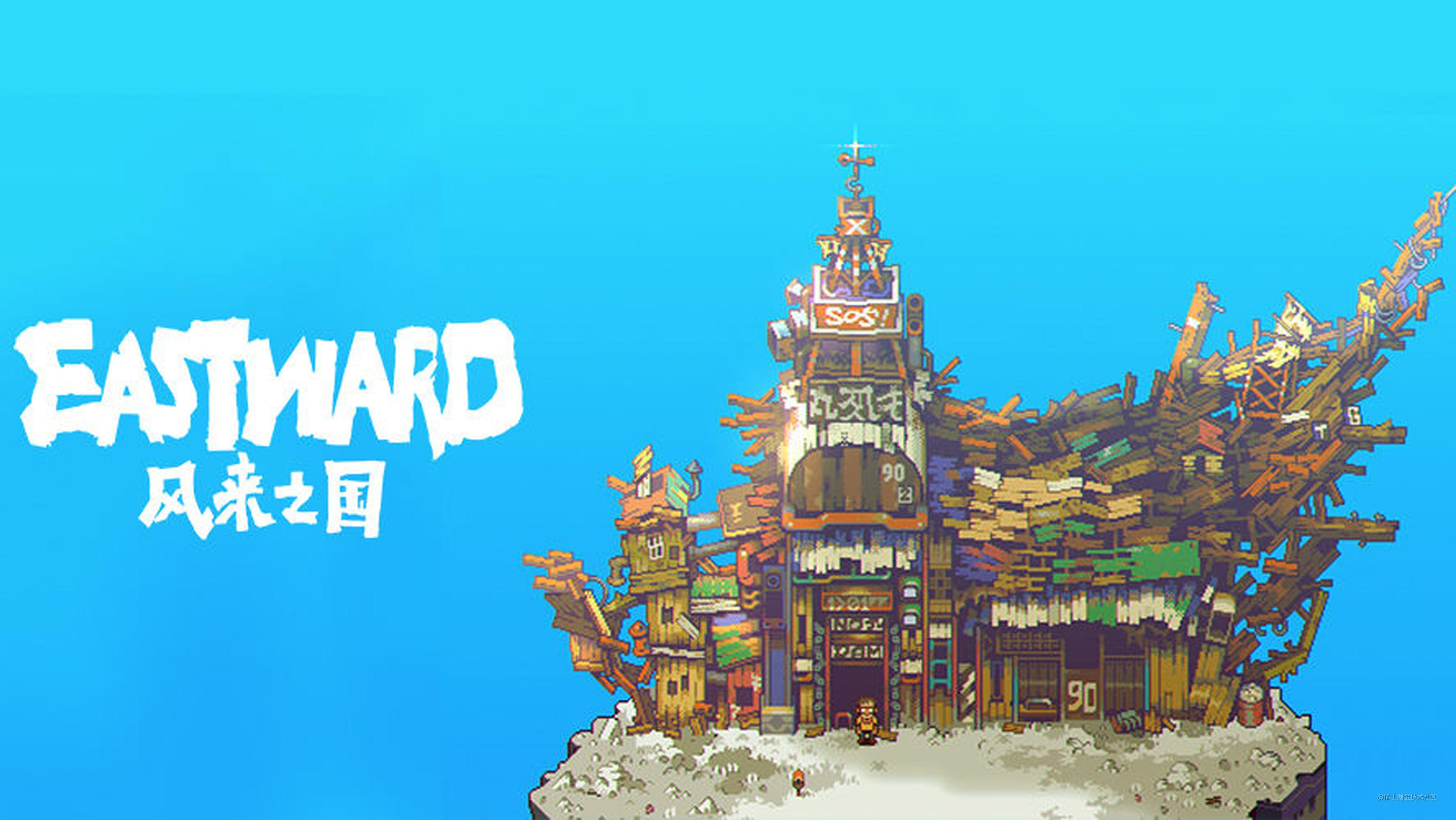# 前言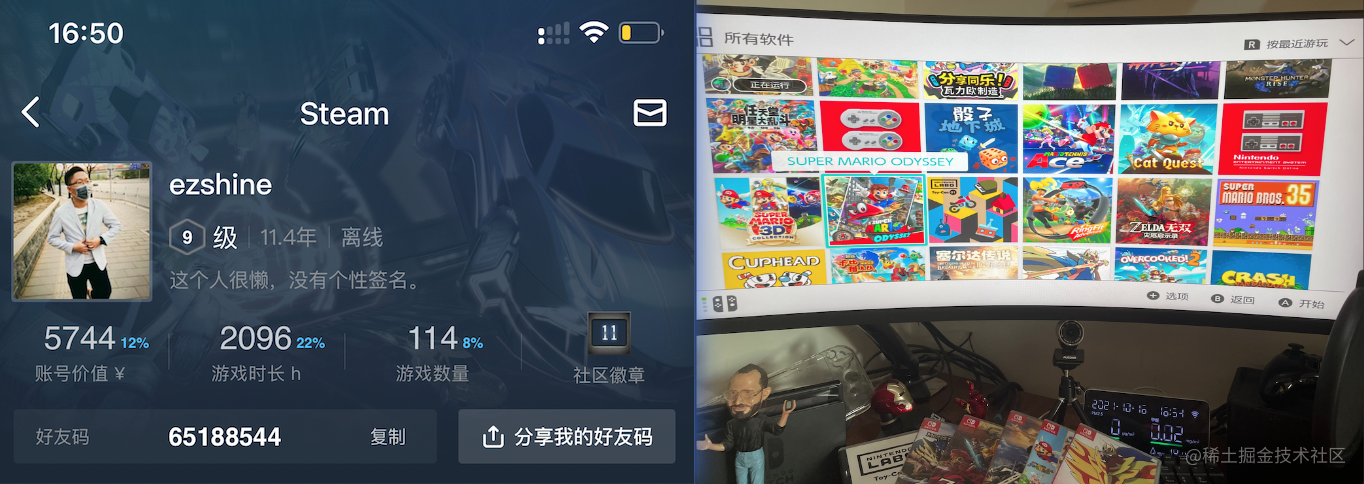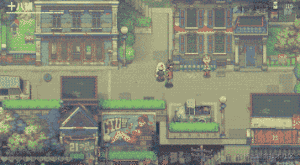• 地图篇：基于`图像`的路墙系统(`GraphicBased`)
• 移动篇：角色和地图相对移动
• 互动篇：和地图上的元素互动

`会不会写完看大家的反响：p`

# 地图篇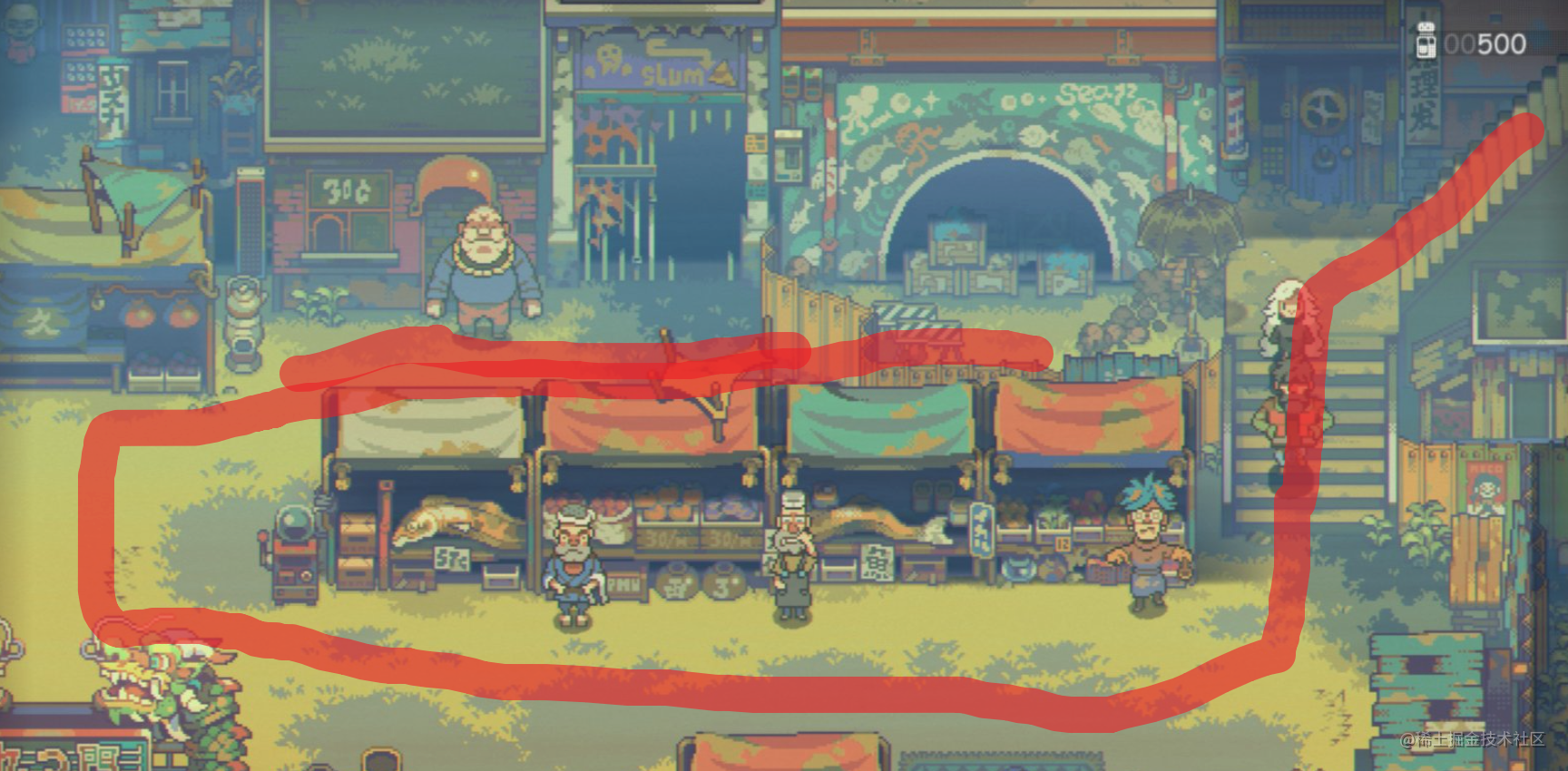• 地板层`角色永远在地板之上`
• 建筑层`会根据角色的位置相互覆盖`

`手绘`了一个游戏地图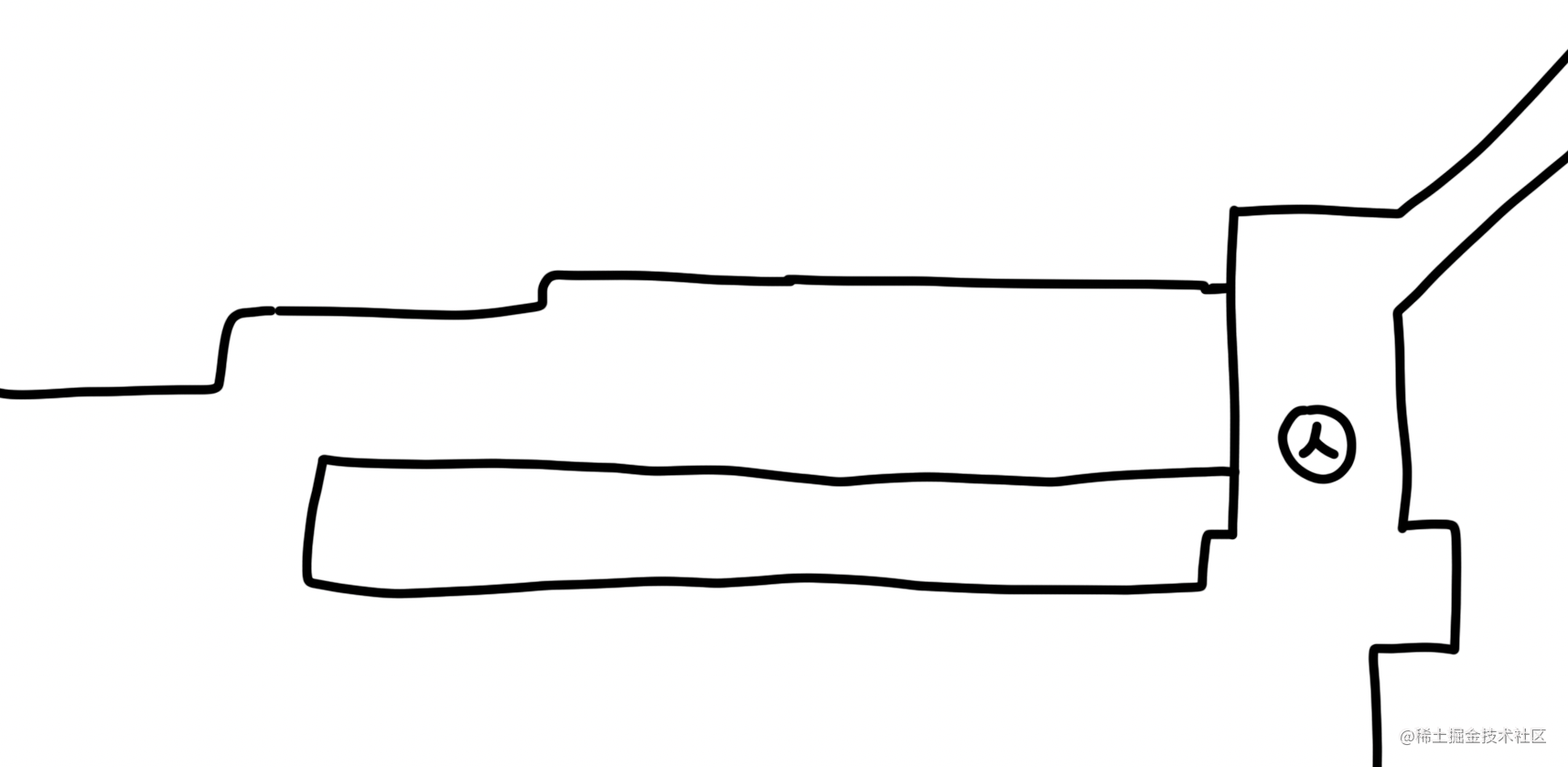# 搭架子

``````<script src="https://cdnjs.cloudflare.com/ajax/libs/pixi.js/5.1.3/pixi.min.js"></script>
<script type="text/javascript">
//初始化PIXI
const app = new PIXI.Application({
backgroundColor:0xffffff
});

//将PIXI的渲染视图添加到网页body中
document.body.appendChild(app.view);

app.renderer.autoResize = true;
app.renderer.resize(window.innerWidth, window.innerHeight);

//加载图片资源
//将图片资源添加到场景
const floor = new PIXI.Sprite(resources.floor.texture);

const player = new PIXI.Sprite(resources.player.texture);

//将玩家图形的原点设置为中心点
player.anchor.x = 0.5;
player.anchor.y = 0.5;

//帧同步事件的回调
});

//上下左右键按下后角色移动
window.onkeydown=function (e) {
if(e.keyCode==37) {
player.x-=2;
}else if(e.keyCode==39){
player.x+=2;
}

if(e.keyCode==38){
player.y-=2;
}else if(e.keyCode==40){
player.y+=2;
}
}
});
}
</script>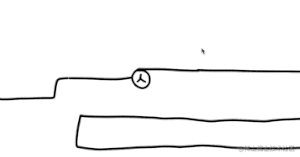``````app.ticker.add(() => {
var nextX = player.x+moveDelta.x;
var nextY = player.y+moveDelta.y;

player.x=nextX;
player.y=nextY;
});

window.onkeyup=function(e){
if(e.keyCode==37||e.keyCode==39) {
moveDelta.x=0;
}else if(e.keyCode==38||e.keyCode==40){
moveDelta.y=0;
}
}
window.onkeydown=function (e) {
if(e.keyCode==37) {
moveDelta.x=-2;
}else if(e.keyCode==39){
moveDelta.x=2;
}

if(e.keyCode==38){
moveDelta.y=-2;
}else if(e.keyCode==40){
moveDelta.y=2;
}
}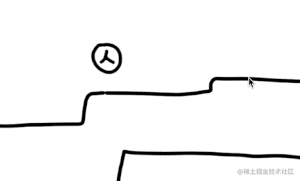# 路墙系统

``````roadarea=[
1,1,1,1,1,1,1,1,
1,0,0,0,0,0,0,1,
1,0,0,0,0,0,0,1,
1,1,1,1,1,1,1,1
]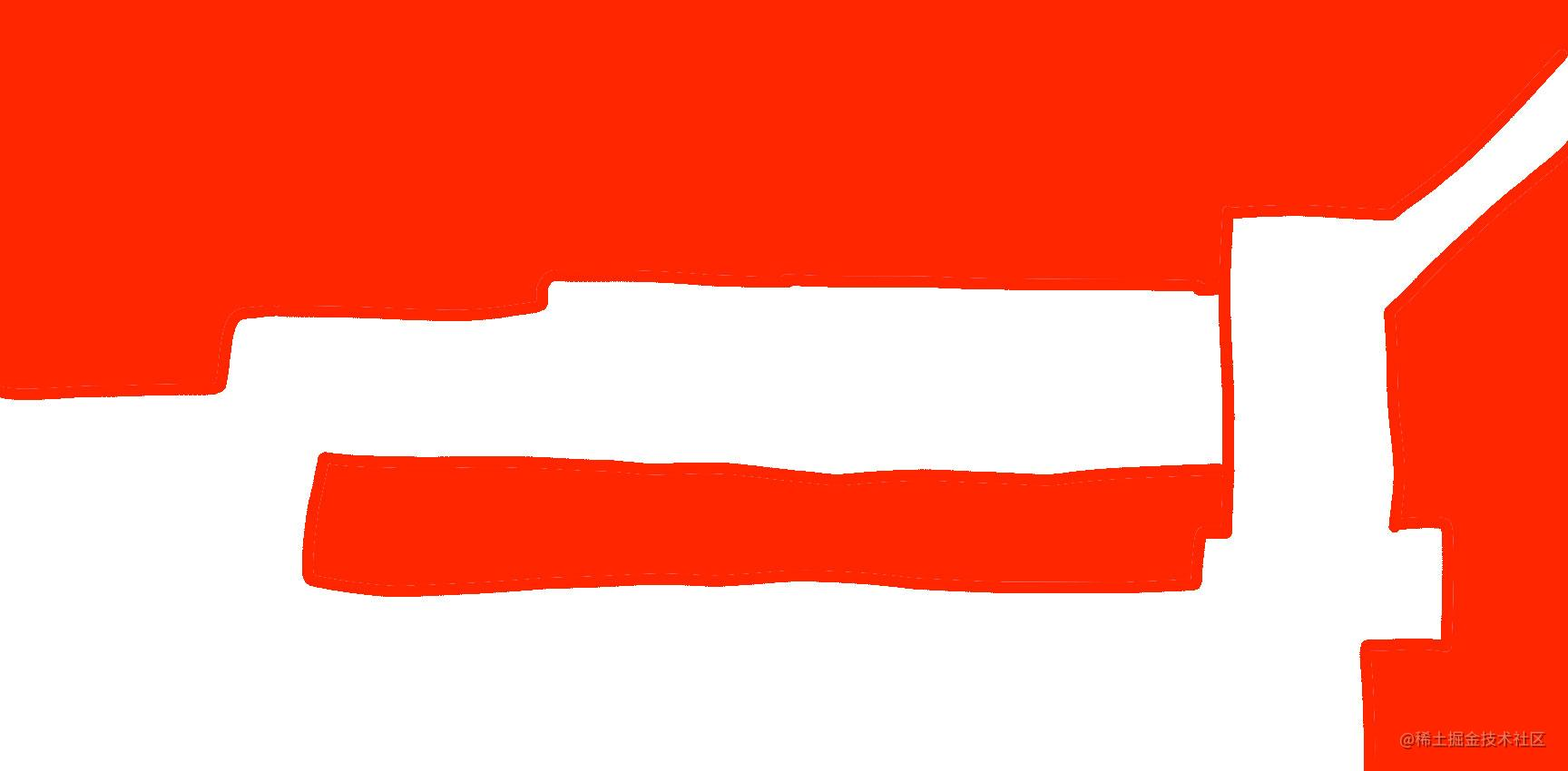``````//将上图中的路墙标识图加载到系统中

//获得路墙标识图的全部像素数组
//格式[r,g,b,a]，所以数组长度为路墙标识图的 宽x高x4

//判断x,y坐标下的像素是否为墙体的标识颜色
function isHitWall(x,y){
var width = 1728;//路墙标识图宽度
var position = (width * y + x) * 4;//还原像素序号

//取出颜色值r,g,b,a
//jpeg格式输出后颜色会略有损失，这里根据实际调整
//就是判断x,y坐标下的颜色是否为红色
if(r==255&&g<50){
console.log("hit the wall");
return true;
}else{
return false;
}
}

var nextX = player.x+moveDelta.x;
var nextY = player.y+moveDelta.y;

//只有在移动的目标坐标下不是墙体颜色时才允许移动
if(!isHitWall(player.x,nextY)){
player.y=nextY;
}

if(!isHitWall(nextX,player.y)){
player.x=nextX;
}
});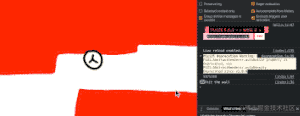# 细节优化

``````// app.stage.addChild(roadarea);

//在判定移动的目标位置时加上操控角色的半径，这里差不多是45
if(!isHitWall(player.x,nextY+45*moveDelta.y)){
player.y=nextY;
}

if(!isHitWall(nextX+45*moveDelta.x,player.y)){
player.x=nextX;
}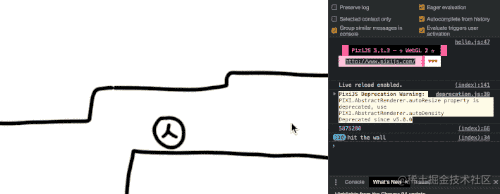• 移动篇：角色和地图相对移动

# 源码

github.com/ezshine/web…

• 哔哩哔哩：`大帅老猿`
• 微信公众号：`大帅老猿`